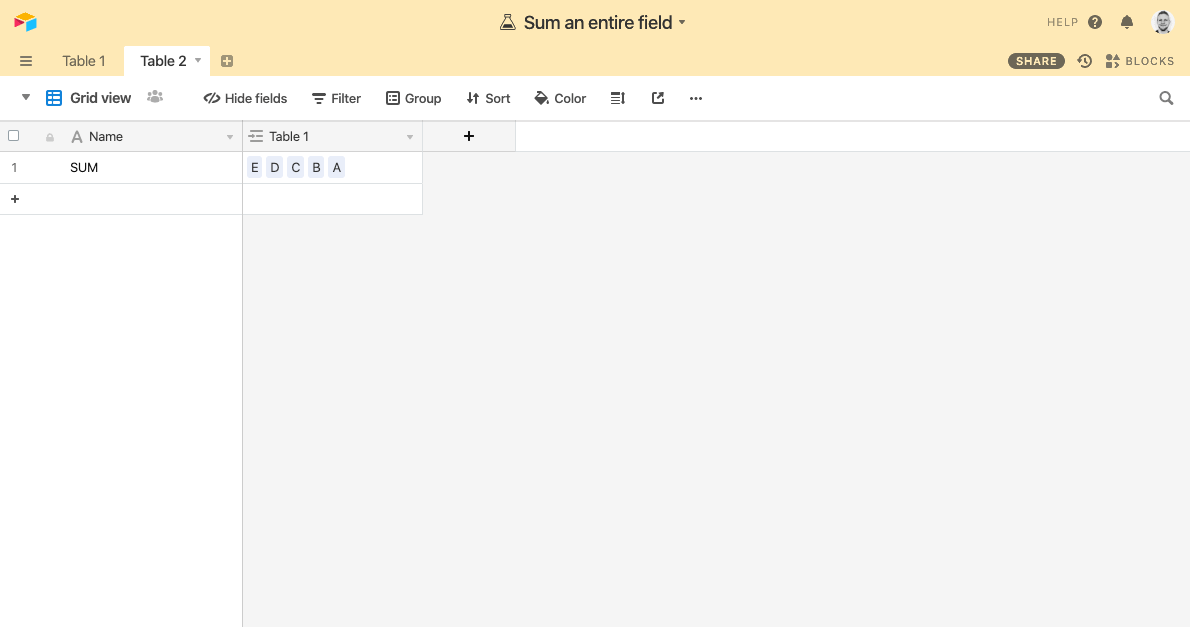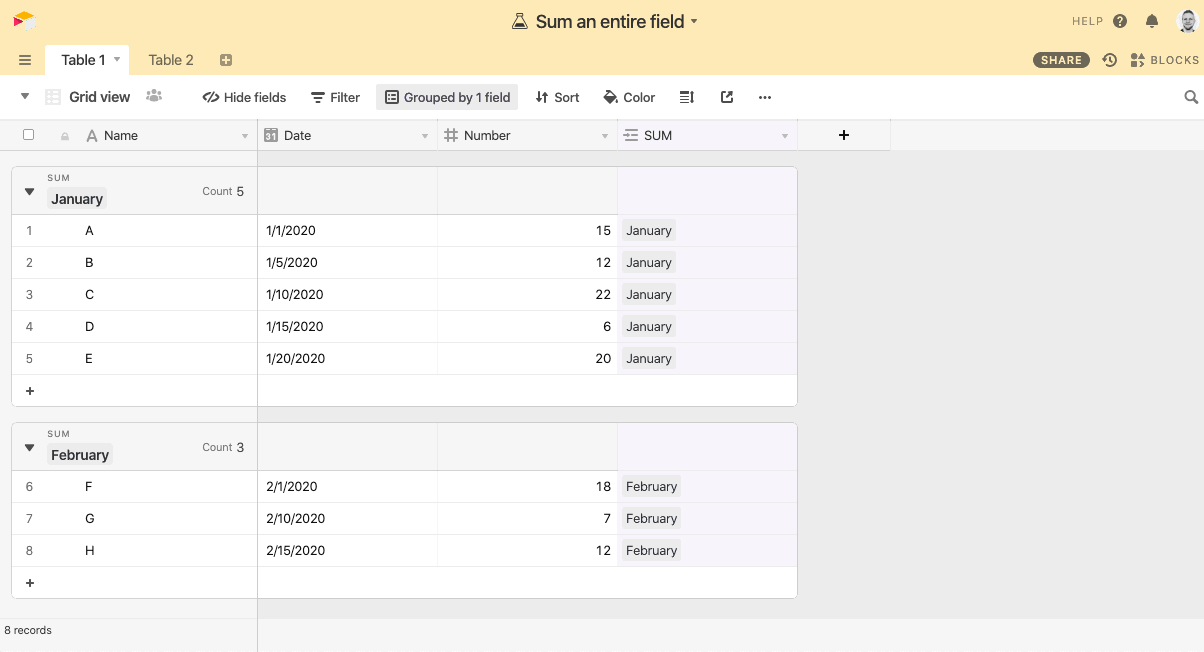• English
• Account
• API
• Integrations
• Enterprise
• Resources
Finding the sum of an entire field
• 31 Oct 2022
• Dark
Light

# Finding the sum of an entire field

• Dark
Light

Article Summary

A core difference between Airtable and a traditional spreadsheet is that Airtable is more than just a spreadsheet - it's also a database. At times, that creates the need for a different approach than a spreadsheet user may be used to.

Finding the sum of a field is one of those scenarios; since formulas apply only to other fields within the same record, you can't create a formula in one record that only references other records in the table. Instead, you can sum an entire field by taking the following steps:

1. Create a new linked record field, and populate every record in the field with the same value. You can do this quickly by using the fill-handle. You can either link this field to an existing table, or create a new table directly from the field settings.1. In the linked table, create a Rollup field that sums the number field with the SUM(values) function.You can use this setup to sum the values of any range of field by adding the same linked record (from Table 2) to each record in Table 1.

You can use the above principle to sum as many fields as you need across your tables. The process will be the same:

1. Create a new record in your linked table (Table 2 in the above example).
2. Link that single record to each of the records you'd like to sum in Table 1.
3. Watch the rollup field referencing Table 2 SUM the values.

## An alternative approach without using a formula

If you only need to quickly see the sum of an entire field - and not use that sum in another formula - you can quickly do so by toggling on the SUM function in the summary bar.